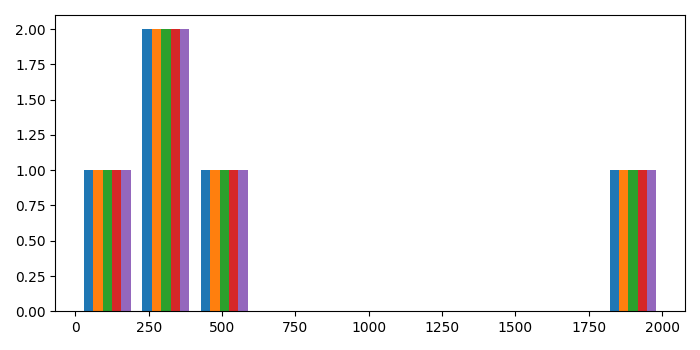# How to make a histogram from a list of data in Matplotlib?

To make a histogram from a list of data in matplotlib, we can take the following steps−

• Create a list of data, i.e., x data points
• Plot a histogram with x data points.
• To display the figure, use show() method.

## Example

from matplotlib import pyplot as plt
plt.rcParams["figure.figsize"] = [7.00, 3.50]
plt.rcParams["figure.autolayout"] = True
x = [[300, 400, 500, 2000, 10], [300, 400, 500, 2000, 10], [300, 400, 500, 2000,
10], [300, 400, 500, 2000, 10], [300, 400, 500, 2000, 10]]
plt.hist(x, 10)
plt.show()

## Output